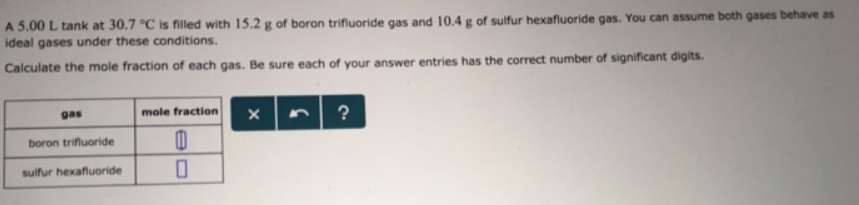# Problem: A 5.00 L tank at 30.7°C is filled with 15.2 g of boron trifluoride gas and 10.4 g of sulfur hexafluoride gas. You can assume both gases behave as ideal gases under these conditions. Calculate the mole fraction of each gas. Be sure each of your answer entries has the correct number of significant digits.

###### FREE Expert Solution
93% (340 ratings)###### Problem Details

A 5.00 L tank at 30.7°C is filled with 15.2 g of boron trifluoride gas and 10.4 g of sulfur hexafluoride gas. You can assume both gases behave as ideal gases under these conditions.

Calculate the mole fraction of each gas. Be sure each of your answer entries has the correct number of significant digits.